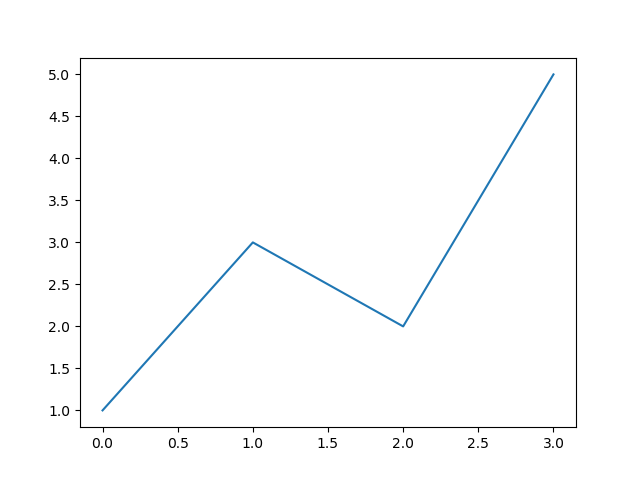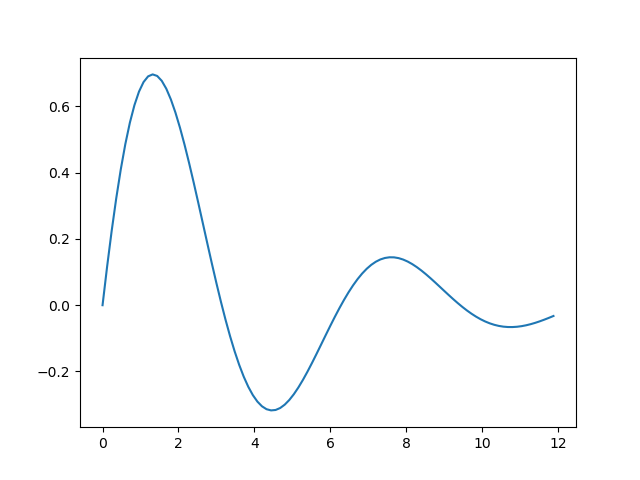# Creating simple plots with Matplotlib

Martin McBride, 2019-03-03
Tags numeric python line plot function plot
Categories matplotlibThe simplest way to create a plot in Matplotlib is to use a list x values and list of y values:

xa = [0, 1, 2, 3]
ya = [1, 3, 2, 5]


We take each pair of (x, y) values in turn. For example, the first x value is 0, the first y value is 1, so the first point is (0, 1). The next point is (1, 3) etc. The complete data set is interpreted as a set of points (0, 1), (1, 3), (2, 2) and (3, 5).

Here is the full code for plotting this data as a line plot:

from matplotlib import pyplot as plt

xa = [0, 1, 2, 3]
ya = [1, 3, 2, 5]

plt.plot(xa, ya)
plt.show()


That is all we need. We import matplotlib.pyplot, shortening the name to plt (this is quite a common thing to do, you will see it frequently in Matplotlib code). Then we plot the data, and show it, which opens a window to display the plot.

Here is the output:As you can see, the graph passes through the points (0, 1), (1, 3), (2, 2) and (3, 5), drawing straight lines between them.

The range of the axes, the colour of the plot, the overall size of the graph, and every other aspect of the plot is set automatically by Matplotlib. This makes it very easy to create a quick graph to look at some data. If you need a graph to include in a document, or on a web page, you might want more control - everything is possible, we will look at that in a later article.

## Plotting a mathematical function

What if we wanted to plot a maths function, for example

$${e^{-x/4}} {\sin x}$$

We do this in a similar way, by calculating a set of points on the curve. However, we usually calculate a larger number of points, very close together. When these points are joined with straight lines, it gives the illusion of a curve.

In this case we will plots the graph over values of x from 0 to 12, and we will plot 100 points to give a smooth looking curve (you can experiment with this, but 100 is a good starting point). Here is how we create the list of x values:

for i in range(100):
xa.append(i*12/100)


This code creates 100 x values in the range 0 to (just less than) 12.

We can now calculate the y values like this:

for x in xa:
ya.append(math.sin(x)*math.exp(-x/4))


The full code to calculate and plot the curve looks like this:

from matplotlib import pyplot as plt
import math

xa = []
ya = []

for i in range(100):
xa.append(i*12/100)

for x in xa:
ya.append(math.sin(x)*math.exp(-x/4))

plt.plot(xa, ya)
plt.show()


Here is the output:We will see in the next article how to use numpy to do this in less code.

Visit the PythonInformer Discussion Forum for numeric Python.

If you found this article useful, you might be interested in the course Introduction to Matplotlib by the same author.

#### Popular tags

2d arrays abstract data type alignment and angle animation arange arc array arrays behavioural pattern bezier curve built-in function callable object chain circle classes clipping close closure cmyk colour combinations comparison operator comprehension context context manager conversion count creational pattern data science data types design pattern device space dictionary drawing duck typing efficiency ellipse else encryption enumerate fill filter font font style for loop function function composition function plot functools game development generativepy tutorial generator geometry gif global variable gradient greyscale higher order function hsl html image image processing imagesurface immutable object in operator index inner function input installing iter iterable iterator itertools join l system lambda function len line linear gradient linspace list list comprehension logical operator lru_cache magic method mandelbrot mandelbrot set map matplotlib monad mutability named parameter numeric python numpy object open operator optimisation optional parameter or pandas partial application path pattern permutations polygon positional parameter print pure function python standard library radial gradient range recipes rectangle recursion reduce repeat rgb rotation roundrect scaling scipy sector segment sequence setup shape singleton slice slicing sound spirograph sprite square str stream string stroke structural pattern subpath symmetric encryption template text text metrics tinkerbell fractal transform translation transparency triangle truthy value tuple turtle unpacking user space vectorisation webserver website while loop zip